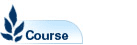Mathematics for Actuarial Studies and Finance 1A - MATH1151

Faculty: Faculty of Science

Course Outline: http://www.maths.unsw.edu.au/

Campus: Sydney

Units of Credit: 6

Indicative Contact Hours per Week: 6

Excluded: ECON1202, ECON2291, MATH1011, MATH1031, MATH1131, MATH1141

Tuition Fee: See Tuition Fee Schedule

Further Information: See Class Timetable

View course information for previous years.

Description

Vectors and vector geometry, linear equations, matrices and matrix algebra, basic input-output linear models, determinants, least squares approximation, probability and statistics. Limits, continuous and differentiable functions, mean value theorem, fundamental theorem of calculus, numerical integration, functions of several variables, introduction to Matlab.

Assumed knowledge: HSC Mathematics Extension 1. Students will be expected to have achieved a combined mark of at least 140 in Mathematics and Mathematics Extension 1 or 180 in Mathematics Extension 1 and Extension 2.